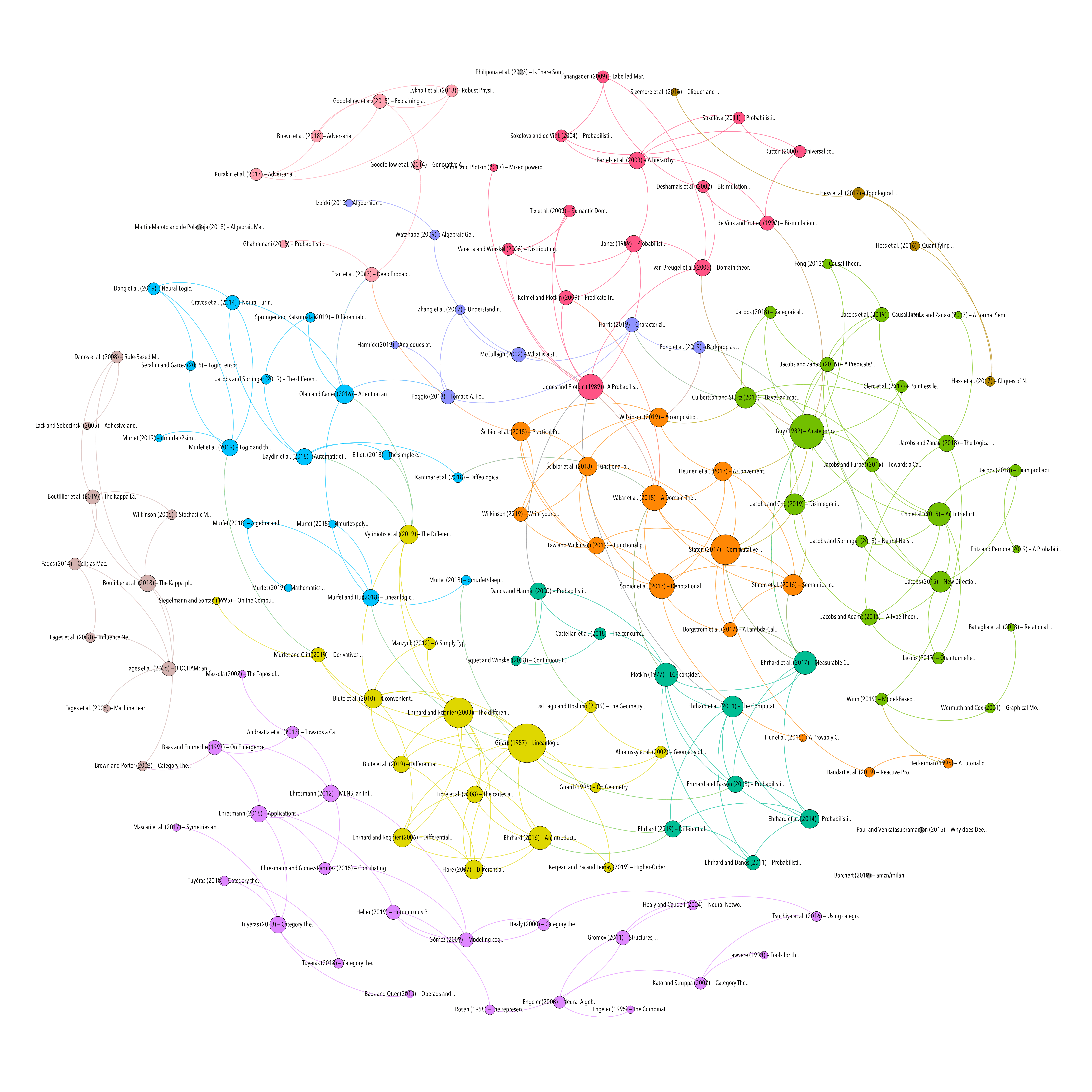MACHINE LEARNING
PROBABILITY & STATISTICS
Topic
Resource type

## Categorical Aspects of Parameter Learning

Resource type
Author/contributor
Title
Categorical Aspects of Parameter Learning
Abstract
Parameter learning is the technique for obtaining the probabilistic parameters in conditional probability tables in Bayesian networks from tables with (observed) data --- where it is assumed that the underlying graphical structure is known. There are basically two ways of doing so, referred to as maximal likelihood estimation (MLE) and as Bayesian learning. This paper provides a categorical analysis of these two techniques and describes them in terms of basic properties of the multiset monad M, the distribution monad D and the Giry monad G. In essence, learning is about the reltionships between multisets (used for counting) on the one hand and probability distributions on the other. These relationsips will be described as suitable natural transformations.
Publication
arXiv:1810.05814 [cs]
Date
2018-10-13
Accessed
2019-11-21T20:38:28Z
Library Catalog
Extra
ZSCC: 0000001 arXiv: 1810.05814
Citation
Jacobs, B. (2018). Categorical Aspects of Parameter Learning. ArXiv:1810.05814 [Cs]. Retrieved from http://arxiv.org/abs/1810.05814
MACHINE LEARNING
PROBABILITY & STATISTICS
Topic
Attachment
Processing time: 0.05 secondsGraph of references (from Zotero to Gephi via Zotnet with this script)